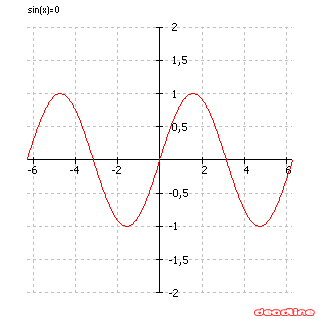# Solve trigonometric equations

Problem: solve a trigonometric equation

Equation to solve: sin(x) = 0

Interval for finding roots: [-2pi, 2pi]

DeadLine can solve trigonometric equations instantly, providing the equation graph and the roots.

Number of roots found: 5

 x1 -6,28318531 x2 -3,14159265 x3 0 x4 3,14159265 x5 6,28318531